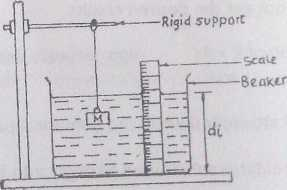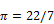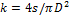Physics Paper 1B, May/June 2012
 Questions: 1 2 3 Main
Weakness/Remedies
Strength

Question 1B

Question 1You have been provided with a beaker, a set of masses and other necessary apparatus.

1. Measure and record the internal diameter D of the beaker
2. Pour some water into the beaker so that it is about half full Measure and record the depth d0 of the water.
3. Suspend a mass M = 50g on the clamp of a retort stand.

Immerse the mass completely into the water as shown in the diagram above.

1. Measure and record the new depth di of the water
2.  Evaluate d = di – d0
3.  Repeat the procedure foe values of M = 100 g, 15O g, 200 g and 250 g
4. In each case measure and record di and also evaluate d = di- do
6. Plot a graph with M on the vertical anti and d on the horizontal axis
7. Deter mine the slope, s, of the graph
8. Evaluate(Take)
9.  State two precautions taken to ensure accurate results

(b)  (i) Define relative density of a body.
(ii)An object weighs 2.7 N in air and 1.2 N when completely immersed in water Calculate the relative density of the body

_____________________________________________________________________________________________________

Majority of the candidates attempted this question and had good and useful results. However, a few of the candidates disregarded the instruction to measure do thus, they could not get the desired results.

1. Evaluation ofwas wrongly done.

Part (b) (i) was well attempted while the numerical part. (b)(ii) was poorly handled Most candidates substituted wrongly into a wrong formula.

1. internal diameter D of beaker measured and recorded to at least 1d.p in cm
2. Initial depth d* of water in the beaker measured and recorded  in

cm to at least 1d.p in cm.

1. Five values of M recorded in gm.
2. Five values of d< measured and recorded to at least 1d.p in cm

and in trend
Trend As M increase di increases

1. Five values of di - do correctly evaluated

1. Composite table showing at least M, dt, and d Candidates are also expected to:
2. plot five points on graph
3. distinguish between the axes
4. chose reasonable scales
5. draw line of best fit
6. evaluate k =4s/nD2
1. State any two of the following precautions in acceptable language.
2. Avoided parallax error in reading the metre rule
3. Avoided splashing of water (Liquid)
4. Avoided object (mass) from touching sides bottom of beaker Metre rule/scale vertically placed

Repeated readings shown on the table

(b) (i) Relative density (R.D) is defined as the ratio of mass ( or weight) of a substance to the mass (weight) of equal volume of water
OR
R D = mass (or weight) of a substance
Mass (or weight) of equal volume of water
OR
R.D = Density of substance Density of water

1. R.D =       Weight in air

Upthrust
RD    =     2.7
2.7- 1.2

=           2.7
1.5
=         1.8